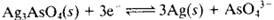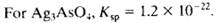### Create an Account

Already have account?

### Forgot Your Password ?

Home / Questions / a Calculate the standard potential for the reaction b Give a schematic representation...

# a Calculate the standard potential for the reaction b Give a schematic representation of a cell with a Silver indicator electrode and an SCE as reference that could be used for determining As

(a) Calculate the standard potential for the reaction(b) Give a schematic representation of a cell with a Silver indicator electrode and an SCE as reference that could be used for determining AsO43.

(c) Derive an equation that relates the measured potential of the cell in (b) to pAsO4 (assume that the junction potential is zero).

(d) Calculate the pAsO4 of a solution that is saturated with Ag3AsO4 and contained in the cell described in (b) if the resulting potential is 0.247 V.

Jun 23 2020 View more View Less

#### Answer (Solved)Subscribe To Get Solution